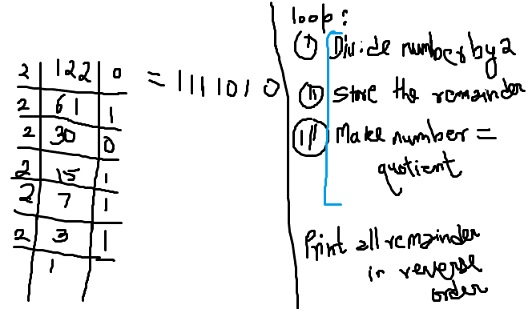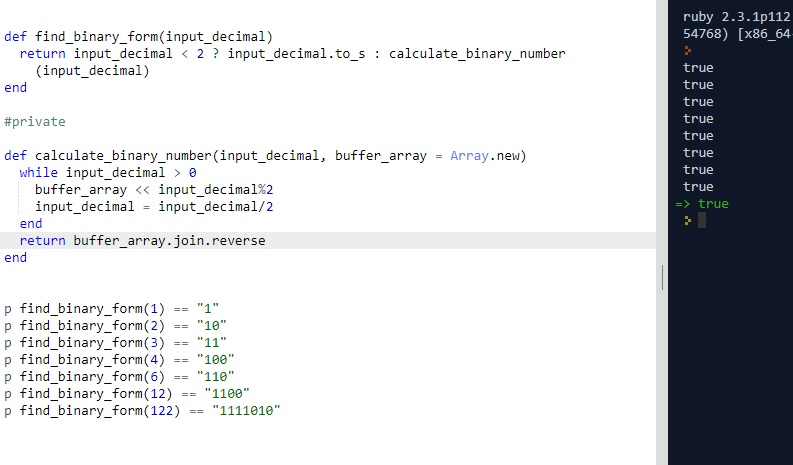# CLEAN CODE: FIND BINARY REPRESENTATION OF A GIVEN NUMBER

By: Saurav

2017-11-12 02:22:00 UTC

Binary Representation
Given a number N >= 0, find its representation in binary.

Example:

if N = 6,

binary form = 110

InterviewBit

This is a very simple problem yet it can be simpler using inbuilt functions in ruby if you know about it. I didn't know about it and I learned something cool and I want to share. :)

The algorithm is same as finding binary representation on a whiteboard/paper.
Figure explains the process:Lets write some tests first:

```p find_binary_form(1) == "1"
p find_binary_form(2) == "10"
p find_binary_form(3) == "11"
p find_binary_form(4) == "100"
p find_binary_form(6) == "110"
p find_binary_form(12) == "1100"
p find_binary_form(122) == "1111010"```

### Attempt 1:

```def find_binary_form(input_decimal)
return input_decimal < 2 ? input_decimal.to_s : calculate_binary_number(input_decimal)
end

#private

def calculate_binary_number(input_decimal, buffer_array = Array.new)
while input_decimal > 0
buffer_array << input_decimal%2
input_decimal = input_decimal/2
end

return buffer_array.join.reverse
end ```All our tests pass and our functions look good as well.

But if we are allowed, here is a one liner answer:

Ruby to_s(base) : Returns a string containing the representation of fix radix base (between 2 and 36).

### Attempt 2:

```def find_binary_form(input_decimal)
return input_decimal.to_s(2)
end
```

sick! ha :)

### Let me know what you think! twitter: sprakash24octlinkedin

Owned & Maintained by Saurav Prakash

If you like what you see, you can help me cover server costs or buy me a cup of coffee though donation :)